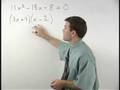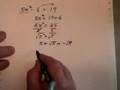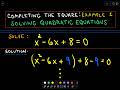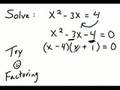Quadratic Equations  ( 0)

Questions

Q. How to solve the quadric equation by completing the square method? ... 3, 001 answer under Quadratic Equations 8 years, 5 months ago

A. For quadratic equations that cannot be solved by factorising, we use a method which can solve ALL quadratic equations called completing the square.We use this later when studying circles in plane... link
8 years, 5 months ago
answer

Q. how to find the roots of general quadratic formulae ... 1, 000 answers under Quadratic Equations 4 years, 1 month ago
answer

Videos more…